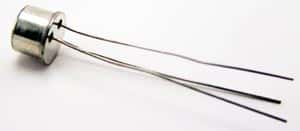# HI13SG 3A 100V TriacManufacturer: Hutson Industries
Part Number: HI13SG
Amps: 3a
Voltage: 100V
Package: TO-5

Price: \$0.90
153 available for immediate delivery
Code: T409PD
Volume Pricing
Quantity Price Each
1-9\$0.90
10-99\$0.85
100+\$0.80
Quantity:

Triacs
Package=TO-5
Crosses to Teccor L2004F61

Specs:
V(DRM) Max.(V)Rep.Pk.Off Volt.=100
I(T(RMS)) Max.(A)On-State Cur.=3.0
@Temp. (�C) (Test Condition)=75
I(TSM) Max. (A)=30
I(GT) Max. (A)=4.0m
V(GT) Max.(V)=2.2
I(H) Max.(A) Holding Current=15m
I(D) Max. (A) Leakage Current=750u
@Temp. (�C) (Test Condition)=100
V(T) Max. (V)=2.2
@I(T) (A) (Test Condition)=10
dv/dt Min. (V/us)=6.0

Manufactured by: Hutson Industries
Part number: HI13SG